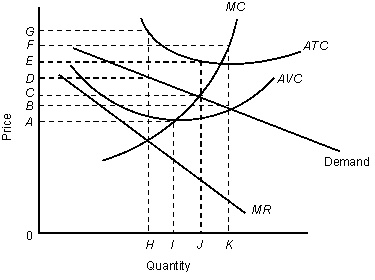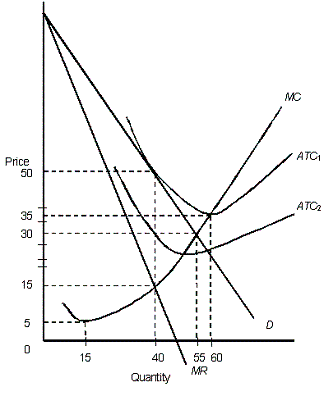/
/
/
11.Which of the following statements about the monopolistically competitive market
Not my Question
Flag Content

# Question : 11.Which of the following statements about the monopolistically competitive market : 1413831

11.Which of the following statements about the monopolistically competitive market in the long run is true?

a.The resources are efficiently utilized.

b.Consumers have a greater variety of products to choose from than under perfectly competitive market conditions.

c.The marginal-revenue curve coincides with the demand curve facing the firm.

d.The firms produce the output level that is less than the output corresponding to the minimum of average total cost.

e.The firms operate in the upward-sloping portion of the long run average cost curve.

12.When the existing firms in a monopolistically competitive industry earn above-normal profit:

a.new firms enter the market, and entry continues until firms earn normal profit.

b.new firms have no incentive to enter the market.

c.new firms have an incentive to enter the market but are legally barred from doing so.

d.they increase their production and lower the price level.

e.their cost structure automatically changes, eliminating the additional profit.

13.As new firms enter a monopolistically competitive industry, the demand for a typical firm’s product will most likely:

a.increase and become less elastic.

b.decrease and become more elastic.

c.increase and become more elastic.

d.decrease and become less elastic.

e.remain unaffected.

14.In long-run equilibrium, the monopolistically competitive firm:

a.will break even.

c.will earn a positive economic profit.

d.will face a perfectly elastic demand curve.

e.will no longer need to engage in non-price competition.

NARRBEGIN: Figure 11.1

The figure given below shows revenue and cost curves of a monopolistically competitive firm.

Figure: 11.1In the figure,

MR: Marginal revenue curve

ATC: Average total cost curve

AVC: Average variable cost curve

MC: Marginal cost curve

NARREND

15.Consider the monopolistically competitive firm described in the Figure 11.1. The profit-maximizing output level and price are, respectively:

a.0 and 0.

b.H and D.

c.I and A.

d.J and C.

e.J and E.

16.According to Figure 11.1, the firm:

a.will shut down temporarily at any price below A.

b.will operate at a price below A as long as it is greater than marginal cost.

c.will shut down at a price below A only in the long run.

d.will break-even at the price level C.

e.will shut down if the price falls below E.

17.According to Figure 11.1, the profit-maximizing firm is making an average:

a.profit on each unit produced equal to the distance BG.

b.loss on each unit produced equal to the distance BG.

c.profit on each unit produced equal to the distance CE.

d.loss on each unit produced equal to the distance DG.

e.loss on each unit produced equal to the distance AC.

18.The short-run equilibrium position for a firm in monopolistic competition is the point at which:

a.price equals average variable cost.

b.marginal revenue equals rising marginal cost.

c.price equals marginal cost.

d.marginal revenue equals average revenue.

e.the firm’s marginal-cost curve intersects its marginal-revenue curve from above.

NARRBEGIN: Figure 11.2

The figure below shows the revenue and cost curves of a monopolistically competitive firm.

Figure: 11.2In the figure,

D: Demand curve

MR: Marginal revenue curve

ATC1 and ATC2: Average total cost curves

MC: Marginal cost curve

NARREND

19.In Figure 11.2, assume that the average total cost of the firm is represented by the curve ATC2. In the long run, we would expect:

a.entry of firms into the market because economic profits exist.

b.exit of firms from the market because the existing firms suffer economic losses.

c.that demand for each firm will increase.

d.the market to become perfectly competitive.

e.the market to become a monopoly.

20.In Figure 11.2, if the market is monopolistically competitive, which quantity represents long-run equilibrium for the firm?

a.15

b.Between 15 and 40

c.40

d.55

e.60

## Solution 5 (1 Ratings )

Solved
Economics 1 Year Ago 74 Views